×#### Thank you for registering.

One of our academic counsellors will contact you within 1 working day.

Click to Chat

1800-1023-196

+91-120-4616500

CART 0

• 0

MY CART (5)

Use Coupon: CART20 and get 20% off on all online Study Material

ITEM
DETAILS
MRP
DISCOUNT
FINAL PRICE
Total Price: Rs.

There are no items in this cart.
Continue Shopping• Complete JEE Main/Advanced Course and Test Series
• OFFERED PRICE: Rs. 15,900
• View Details

```Chapter 15: Properties of Triangles Exercise – 15.2

Question: 1

Two angles of a triangle are of measures 150° and 30°. Find the measure of the third angle.

Solution:

Let the third angle be x

Sum of all the angles of a triangle = 180°

105° + 30° + x = 180°

135° + x = 180°

x = 180° – 135°

x = 45°

Therefore the third angle is 45°

Question: 2

One of the angles of a triangle is 130°, and the other two angles are equal. What is the measure of each of these equal angles?

Solution:

Let the second and third angle be x

Sum of all the angles of a triangle = 180°

130° + x + x = 180°

130° + 2x = 180°

2x = 180°– 130°

2x = 50°

x = 50/2

x = 25°

Therefore the two other angles are 25° each

Question: 3

The three angles of a triangle are equal to one another. What is the measure of each of the angles?

Solution:

Let the each angle be x

Sum of all the angles of a triangle =180°

x + x + x = 180°

3x = 180°

x = 180/3

x = 60°

Therefore angle is 60° each

Question: 4

If the angles of a triangle are in the ratio 1: 2: 3, determine three angles.

Solution:

If angles of the triangle are in the ratio 1: 2: 3 then take first angle as ‘x’, second angle as ‘2x’ and third angle as ‘3x’

Sum of all the angles of a triangle=180°

x + 2x + 3x = 180°

6x = 180°

x = 180/6

x = 30°

2x = 30° × 2 = 60°

3x = 30° × 3 = 90°

Therefore the first angle is 30°, second angle is 60° and third angle is 90°

Question: 5

The angles of a triangle are (x − 40) °, (x − 20) ° and (1/2 − 10) °. Find the value of x.

Solution:Question: 6

The angles of a triangle are arranged in ascending order of magnitude. If the difference between two consecutive angles is 10°. Find the three angles.

Solution:

Let the first angle be x

Second angle be x + 10°

Third angle be x + 10° + 10°

Sum of all the angles of a triangle = 180°

x + x + 10° + x + 10° +10° = 180°

3x + 30 = 180

3x = 180 - 30

3x = 150

x = 150/3

x = 50°

First angle is 50°

Second angle x + 10° = 50 + 10 = 60°

Third angle x + 10° +10° = 50 + 10 + 10 = 70°

Question: 7

Two angles of a triangle are equal and the third angle is greater than each of those angles by 30°. Determine all the angles of the triangle

Solution:

Let the first and second angle be x

The third angle is greater than the first and second by 30° = x + 30°

The first and the second angles are equal

Sum of all the angles of a triangle = 180°

x + x + x + 30° = 180°

3x + 30 = 180

3x = 180 - 30

3x = 150

x = 150/3

x = 50°

Third angle = x + 30° = 50° + 30° = 80°

The first and the second angle is 50° and the third angle is 80°

Question: 8

If one angle of a triangle is equal to the sum of the other two, show that the triangle is a right triangle.

Solution:

One angle of a triangle is equal to the sum of the other two

x = y + z

Let the measure of angles be x, y, z

x + y + z = 180°

x + x = 180°

2x = 180°

x = 180/2

x = 90°

If one angle is 90° then the given triangle is a right angled triangle

Question: 9

If each angle of a triangle is less than the sum of the other two, show that the triangle is acute angled.

Solution:

Each angle of a triangle is less than the sum of the other two

Measure of angles be x, y and z

x > y + z

y < x + z

z < x + y

Therefore triangle is an acute triangle

Question: 10

In each of the following, the measures of three angles are given. State in which cases the angles can possibly be those of a triangle:

(i) 63°, 37°, 80°

(ii) 45°, 61°, 73°

(iii) 59°, 72°, 61°

(iv) 45°, 45°, 90°

(v) 30°, 20°, 125°

Solution:

(i) 63°, 37°, 80° = 180°

Angles form a triangle

(ii) 45°, 61°, 73° is not equal to 180°

Therefore not a triangle

(iii) 59°, 72°, 61° is not equal to 180°

Therefore not a triangle

(iv) 45°, 45°, 90° = 180°

Angles form a triangle

(v) 30°, 20°, 125° is not equal to 180°

Therefore not a triangle

Question: 11

The angles of a triangle are in the ratio 3: 4: 5. Find the smallest angle

Solution:

Given that

Angles of a triangle are in the ratio: 3: 4: 5

Measure of the angles be 3x, 4x, 5x

Sum of the angles of a triangle =180°

3x + 4x + 5x = 180°

12x = 180°

x = 180/12

x = 15°

Smallest angle = 3x

=3 × 15°

= 45°

Question: 12

Two acute angles of a right triangle are equal. Find the two angles.

Solution:

Given acute angles of a right angled triangle are equal

Right triangle: whose one of the angle is a right angle

Measured angle be x, x, 90°

x + x + 180°= 180°

2x = 90°

x = 90/2

x = 45°

The two angles are 45° and 45°

Question: 13

One angle of a triangle is greater than the sum of the other two. What can you say about the measure of this angle? What type of a triangle is this?

Solution:

Angle of a triangle is greater than the sum of the other two

Measure of the angles be x, y, z

x > y + z  or

y > x + z   or

z > x + y

x or y or z > 90° which is obtuse

Therefore triangle is an obtuse angle

Question: 14

AC, AD and AE are joined. Find

∠FAB + ∠ABC + ∠BCD + ∠CDE + ∠DEF + ∠EFA

∠FAB + ∠ABC + ∠BCD + ∠CDE + ∠DEF + ∠EFA

Solution:

We know that sum of the angles of a triangle is 180°Therefore in ∆ABC, we have

∠CAB + ∠ABC + ∠BCA = 180° — (i)

In ∆ACD, we have

∠DAC + ∠ACD + ∠CDA = 180° — (ii)

In ∆AEF, we have

∠FAE + ∠AEF + ∠EFA = 180° — (iv)

Adding (i), (ii), (iii), (iv) we get

∠CAB + ∠ABC + ∠BCA + ∠DAC + ∠ACD + ∠CDA + ∠EAD + ∠ADE + ∠DEA + ∠FAE + ∠AEF +∠EFA = 720°

Therefore ∠FAB + ∠ABC + ∠BCD + ∠CDE + ∠DEF + ∠EFA = 720°

Question: 15

Find x, y, z (whichever is required) from the figures given below: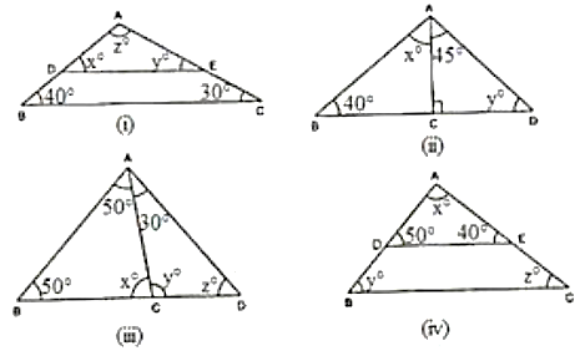Solution:

(i) In ∆ABC and ∆ADE we have:

x = 40°

∠AED = ∠ACB (corresponding angles)

y = 30°

We know that the sum of all the three angles of a triangle is equal to 180°

x + y + z = 180° (Angles of ∆ADE)

Which means: 40° + 30° + z = 180°

z = 180° - 70°

z = 110°

Therefore, we can conclude that the three angles of the given triangle are 40°, 30° and 110°.

(ii) We can see that in ∆ADC, ∠ADC is equal to 90°.

We also know that the sum of all the angles of a triangle is equal to 180°.

Which means: 45° + 90° + y = 180° (Sum of the angles of ∆ADC)

135° + y = 180°

y = 180° – 135°.

y = 45°.

We can also say that in ∆ABC, ∠ABC + ∠ACB + ∠BAC is equal to 180°.

(Sum of the angles of ∆ABC)

40° + y + (x + 45°) = 180°

40° + 45° + x + 45° = 180°  (y = 45°)

x = 180° –130°

x = 50°

Therefore, we can say that the required angles are 45° and 50°.

(iii) We know that the sum of all the angles of a triangle is equal to 180°.

Therefore, for △ABD:

∠ABD +∠ADB + ∠BAD = 180° (Sum of the angles of ∆ABD)

50° + x + 50° = 180°

100° + x = 180°

x = 180° – 100°

x = 80°

For ∆ABC:

∠ABC + ∠ACB + ∠BAC = 180° (Sum of the angles of ∆ABC)

50° + z + (50° + 30°) = 180°

50° + z + 50° + 30° = 180°

z = 180° – 130°

z = 50°

Using the same argument for ∆ADC:

∠ADC + ∠ACD + ∠DAC = 180° (Sum of the angles of ∆ADC)

y +z + 30° =180°

y + 50° + 30° = 180° (z = 50°)

y = 180° – 80°

y = 100°

Therefore, we can conclude that the required angles are 80°, 50° and 100°.

(iv) In ∆ABC and ∆ADE we have:

y = 50°

Also, ∠AED = ∠ACB  (Corresponding angles)

z = 40°

We know that the sum of all the three angles of a triangle is equal to 180°.

Which means: x + 50° + 40° = 180° (Angles of ∆ADE)

x = 180° – 90°

x = 90°

Therefore, we can conclude that the required angles are 50°, 40° and 90°.

Question: 16

If one angle of a triangle is 60° and the other two angles are in the ratio 1: 2, find the angles

Solution:

We know that one of the angles of the given triangle is 60°. (Given)

We also know that the other two angles of the triangle are in the ratio 1: 2.

Let one of the other two angles be x.

Therefore, the second one will be 2x.

We know that the sum of all the three angles of a triangle is equal to 180°.

60° + x + 2x = 180°

3x = 180° – 60°

3x = 120°

x = 120/3

x = 40°

2x = 2 × 40

2x = 80°

Hence, we can conclude that the required angles are 40° and 80°.

Question: 17

It one angle of a triangle is 100° and the other two angles are in the ratio 2: 3. find the angles.

Solution:

We know that one of the angles of the given triangle is 100°.

We also know that the other two angles are in the ratio 2: 3.

Let one of the other two angles be 2x.

Therefore, the second angle will be 3x.

We know that the sum of all three angles of a triangle is 180°.

100° + 2x + 3x = 180°

5x = 180° – 100°

5x = 80°

x = 80/5

2x = 2 ×16

2x = 32°

3x = 3×16

3x = 48°

Thus, the required angles are 32° and 48°.

Question: 18

In △ABC, if 3∠A = 4∠B = 6∠C, calculate the angles.

Solution:

We know that for the given triangle, 3∠A = 6∠C

∠A = 2∠C — (i)

We also know that for the same triangle, 4∠B = 6∠C

∠B = (6/4)∠C  — (ii)

We know that the sum of all three angles of a triangle is 180°.

Therefore, we can say that:

∠A + ∠B + ∠C = 180° (Angles of ∆ABC) — (iii)

On putting the values of ∠A and ∠B in equation (iii), we get:

2∠C + (6/4)∠C +∠C = 180°

(18/4) ∠C = 180°

∠C = 40°

From equation (i), we have:

∠A = 2∠C = 2 × 40

∠A = 80°

From equation (ii), we have:

∠B = (6/4)∠C = (6/4) × 40°

∠B = 60°

∠A = 80°, ∠B = 60°, ∠C = 40°

Therefore, the three angles of the given triangle are 80°, 60°, and 40°.

Question: 19

Is it possible to have a triangle, in which

(i) Two of the angles are right?

(ii) Two of the angles are obtuse?

(iii) Two of the angles are acute?

(iv) Each angle is less than 60°?

(v) Each angle is greater than 60°?

(vi) Each angle is equal to 60°

Solution:

(i) No, because if there are two right angles in a triangle, then the third angle of the triangle must be zero, which is not possible.

(ii) No, because as we know that the sum of all three angles of a triangle is always 180°. If there are two obtuse angles, then their sum will be more than 180°, which is not possible in case of a triangle.

(iii) Yes, in right triangles and acute triangles, it is possible to have two acute angles.

(iv) No, because if each angle is less than 60°, then the sum of all three angles will be less than 180°, which is not possible in case of a triangle.

Proof:

Let the three angles of the triangle be ∠A, ∠B and ∠C.

As per the given information,

∠A < 60°  … (i)

∠B< 60°   … (ii)

∠C < 60°   … (iii)

On adding (i), (ii) and (iii), we get:

∠A + ∠B + ∠C < 60°+ 60°+ 60°

∠A + ∠B + ∠C < 180°

We can see that the sum of all three angles is less than 180°, which is not possible for a triangle.

Hence, we can say that it is not possible for each angle of a triangle to be less than 60°.

(v) No, because if each angle is greater than 60°, then the sum of all three angles will be greater than 180°, which is not possible.

Proof:

Let the three angles of the triangle be ∠A, ∠B and ∠C. As per the given information,

∠A > 60°  … (i)

∠B > 60°   … (ii)

∠C >  60°   … (iii)

On adding (i), (ii) and (iii), we get:

∠A + ∠B + ∠C > 60°+ 60°+ 60°

∠A + ∠B + ∠C > 180°

We can see that the sum of all three angles of the given triangle are greater than 180°, which is not possible for a triangle.

Hence, we can say that it is not possible for each angle of a triangle to be greater than 60°.

(vi) Yes, if each angle of the triangle is equal to 60°, then the sum of all three angles will be 180° , which is possible in case of a triangle.

Proof:

Let the three angles of the triangle be ∠A, ∠B and ∠C. As per the given information,

∠A = 60°  … (i)

∠B = 60°   …(ii)

∠C = 60°   … (iii)

On adding (i), (ii) and (iii), we get:

∠A + ∠B + ∠C = 60°+ 60°+ 60°

∠A + ∠B + ∠C =180°

We can see that the sum of all three angles of the given triangle is equal to 180°, which is possible in case of a triangle. Hence, we can say that it is possible for each angle of a triangle to be equal to 60°.

Question: 20

In ∆ABC, ∠A = 100°, AD bisects ∠A and AD perpendicular BC. Find ∠B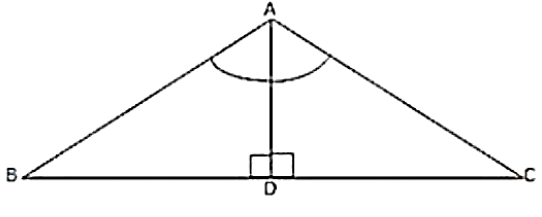Solution:

Consider ∆ABD

We know that the sum of all three angles of a triangle is 180°.

Thus,

∠ABD + ∠BAD + ∠ADB = 180° (Sum of angles of ∆ABD)

Or,

∠ABD + 50° + 90° = 180°

∠ABD =180° – 140°

∠ABD = 40°

Question: 21

In ∆ABC, ∠A = 50°, ∠B = 100° and bisector of ∠C meets AB in D. Find the angles of the triangles ADC and BDC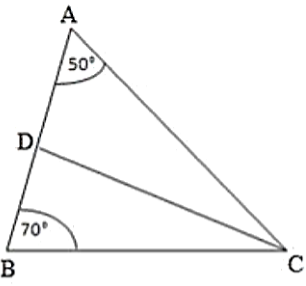Solution:

We know that the sum of all three angles of a triangle is equal to 180°.

Therefore, for the given △ABC, we can say that:

∠A + ∠B + ∠C = 180° (Sum of angles of ∆ABC)

50° + 70° + ∠C = 180°

∠C= 180° –120°

∠C = 60°

∠ACD = ∠BCD =∠C2 (CD bisects ∠C and meets AB in D. )

∠ACD = ∠BCD = 60/2= 30°

Using the same logic for the given ∆ACD, we can say that:

∠DAC + ∠ACD + ∠ADC = 180°

50° + 30° + ∠ADC = 180°

If we use the same logic for the given ∆BCD, we can say that

∠DBC + ∠BCD + ∠BDC = 180°

70° + 30° + ∠BDC = 180°

∠BDC = 180° – 100°

∠BDC = 80°

Thus,

For ∆ADC: ∠A = 50°, ∠D = 100° ∠C = 30°

∆BDC: ∠B = 70°, ∠D = 80° ∠C = 30°

Question: 22

In ∆ABC, ∠A = 60°, ∠B = 80°, and the bisectors of ∠B and ∠C,  meet at O. Find

(i) ∠C

(ii) ∠BOCSolution:

We know that the sum of all three angles of a triangle is 180°.

Hence, for △ABC, we can say that:

∠A + ∠B + ∠C = 180° (Sum of angles of ∆ABC)

60° + 80° + ∠C= 180°.

∠C = 180° – 140°

∠C = 140°.

For △OBC,

∠OBC = ∠B2 = 80/2 (OB bisects ∠B)

∠OBC = 40°

∠OCB =∠C2 = 40/2 (OC bisects ∠C)

∠OCB = 20°

If we apply the above logic to this triangle, we can say that:

∠OCB + ∠OBC + ∠BOC = 180° (Sum of angles of ∆OBC)

20° + 40° + ∠BOC = 180°

∠BOC = 180° – 60°

∠BOC = 120°

Question: 23

The bisectors of the acute angles of a right triangle meet at O. Find the angle at O between the two bisectors.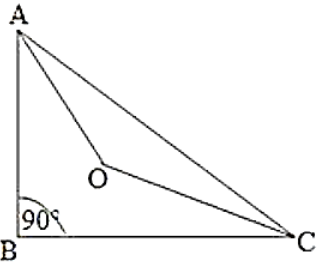Solution:

We know that the sum of all three angles of a triangle is 180°.

Hence, for ∆ABC, we can say that:

∠A + ∠B + ∠C = 180°

∠A + 90° + ∠C = 180°

∠A + ∠C = 180° – 90°

∠A + ∠C = 90°

For ∆OAC:

∠OAC = ∠A2           (OA bisects LA)

∠OCA   = ∠C2         (OC bisects LC)

On applying the above logic to △OAC, we get:

∠AOC + ∠OAC + ∠OCA = 180°    (Sum of angles of ∆AOC)

∠AOC + ∠A2 + ∠C2 = 180°

∠AOC + ∠A + ∠C2 = 180°

∠AOC + 90/2 = 180°

∠AOC = 180° – 45°

∠AOC = 135°

Question: 24

In ∆ABC, ∠A = 50° and BC is produced to a point D. The bisectors of ∠ABC and ∠ACD meet at E. Find ∠E.Solution:

In the given triangle,

∠ACD = ∠A + ∠B. (Exterior angle is equal to the sum of two opposite interior angles.)

We know that the sum of all three angles of a triangle is 180°.

Therefore, for the given triangle, we can say that: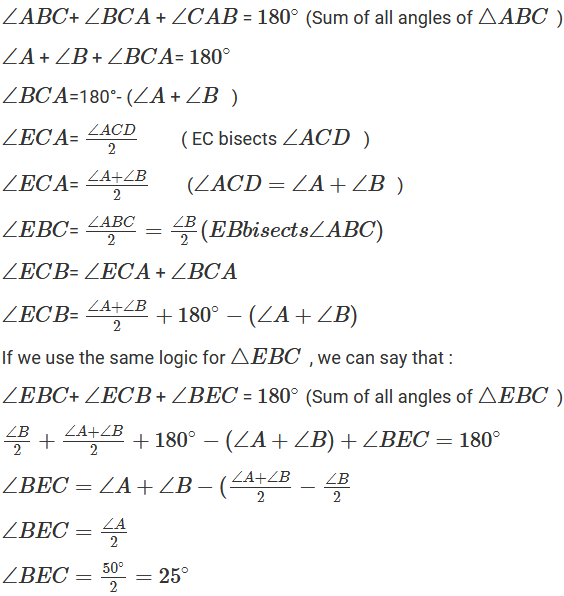Question: 25

In ∆ABC, ∠B = 60°, ∠C = 40°, AL perpendicular BC and AD bisects ∠A such that L and D lie on side BC. Find ∠LAD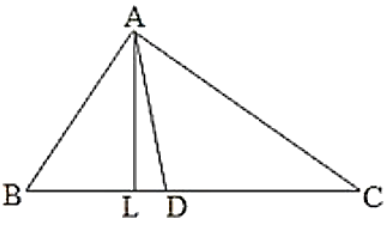Solution:

We know that the sum of all angles of a triangle is 180°

Therefore, for ∆ABC, we can say that: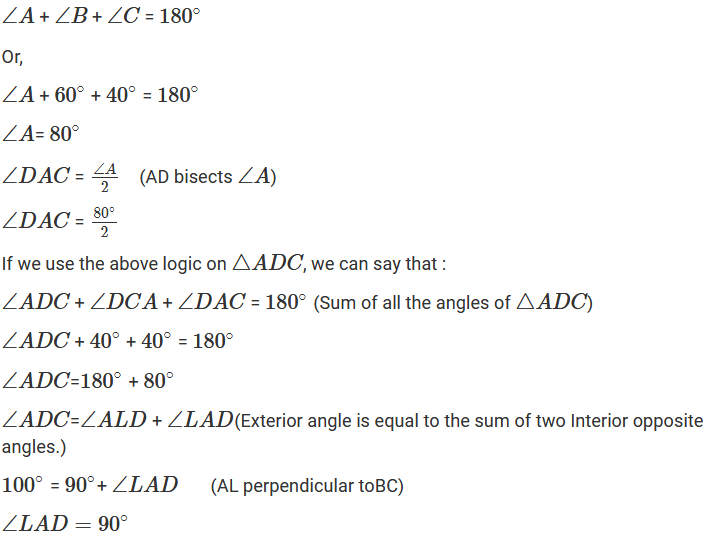Question: 26

Line segments AB and CD intersect at O such that AC perpendicular DB. It ∠CAB = 35° and ∠CDB = 55°. Find ∠BOD.Solution:

We know that AC parallel to BD and AB cuts AC and BD at A and B, respectively.

∠CAB = ∠DBA (Alternate interior angles)

∠DBA = 35°

We also know that the sum of all three angles of a triangle is 180°.

Hence, for △OBD, we can say that:

∠DBO + ∠ODB + ∠BOD = 180°

35° + 55° + ∠BOD = 180° (∠DBO = ∠DBA and ∠ODB = ∠CDB)

∠BOD = 180° - 90°

∠BOD = 90°

Question: 27

In Figure, ∆ABC is right angled at A, Q and R are points on line BC and P is a point such that QP perpendicular to AC and RP perpendicular to AB. Find ∠PSolution:

In the given triangle, AC parallel to QP and BR cuts AC and QP at C and Q, respectively.

∠QCA = ∠CQP (Alternate interior angles)

Because RP parallel to AB and BR cuts AB and RP at B and R, respectively,

∠ABC = ∠PRQ (alternate interior angles).

We know that the sum of all three angles of a triangle is 180°.

Hence, for ∆ABC, we can say that:

∠ABC + ∠ACB + ∠BAC = 180°

∠ABC + ∠ACB + 90° = 180° (Right angled at A)

∠ABC + ∠ACB = 90°

Using the same logic for △PQR, we can say that:

∠PQR + ∠PRQ + ∠QPR = 180°

∠ABC + ∠ACB + ∠QPR = 180° (∠ABC = ∠PRQ and ∠QCA = ∠CQP)

Or,

90°+ ∠QPR =180° (∠ABC+ ∠ACB = 90°)

∠QPR = 90°
```### Course Features

• 728 Video Lectures
• Revision Notes
• Previous Year Papers
• Mind Map
• Study Planner
• NCERT Solutions
• Discussion Forum
• Test paper with Video Solution